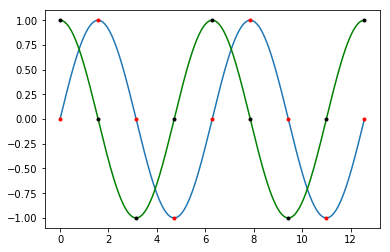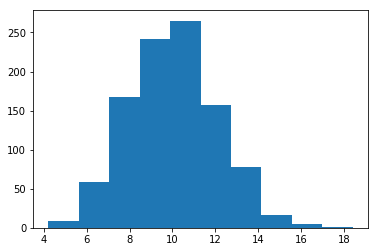# Python fundamentals¶

This notebook gives the basics of Python 3.

The best resource for beginners is The Python Tutorial.

## Examples¶

You can quickly do lots of things in Python, such as manipulate an image.

Excellent packages exist for almost anything reasonable a computer can do.

In :
# You need to install Pillow for this first: conda install -c anaconda pillow

import urllib.request
import io
import PIL.Image

URL = 'http://www.gmit.ie/sites/all/themes/gmitpublic/images/gmit_logo.png'

with urllib.request.urlopen(URL) as url:
imagefile = io.BytesIO(url.read())
image = PIL.Image.open(imagefile)
imagebw = image.convert('L')

display(image, imagebw)Many plotting packages exist, such as matplotlib.

In :
%matplotlib inline

import numpy as np
import matplotlib.pyplot as pl

x1 = np.linspace(0, 4 * np.pi, 1000)
y1 = np.sin(x1)
y2 = np.cos(x1)

x2 = np.linspace(0, 4 * np.pi, 9)
y3 = np.sin(x2)
y4 = np.cos(x2)

pl.plot(x1, y1)
pl.plot(x2, y3, 'r.')
pl.plot(x1, y2, 'g')
pl.plot(x2, y4, 'k.')

pl.show()Python is an excellent choice for data analytics.

In :
%matplotlib inline

import numpy as np
import matplotlib.pyplot as pl

pl.hist(np.random.normal(10, 2, 1000))
pl.show()## Philosophy¶

One reason Python is popular is because of its philosophy.

There's an Easter egg you can find as follows.

In :
import this

The Zen of Python, by Tim Peters

Beautiful is better than ugly.
Explicit is better than implicit.
Simple is better than complex.
Complex is better than complicated.
Flat is better than nested.
Sparse is better than dense.
Readability counts.
Special cases aren't special enough to break the rules.
Although practicality beats purity.
Errors should never pass silently.
Unless explicitly silenced.
In the face of ambiguity, refuse the temptation to guess.
There should be one-- and preferably only one --obvious way to do it.
Although that way may not be obvious at first unless you're Dutch.
Now is better than never.
Although never is often better than *right* now.
If the implementation is hard to explain, it's a bad idea.
If the implementation is easy to explain, it may be a good idea.
Namespaces are one honking great idea -- let's do more of those!


## Offside rule¶

Python uses the offside rule instead of curly braces and semi-colons.

Its purpose is to improve the readability of code.

## Comments¶

Comments come after a hash character, #.

In :
# This section of code
# does
#### absolutely nothing.

In :
print(1) # Print the number 1.

1


## Variables¶

Python doesn't require the declaration of variables or their type.

You can just start using a new variable, and Python won't complain.

Whole number literals, like 123, are of type int.

In :
i = 123
i

Out:
123

You can assign two or more variables at once using commas.

In :
m, n = 10, 11
print(m, n)

10 11


So, you can do this:

In :
a = 1
b = 2

print(a, b)

a, b = b, a

print(a, b)

1 2
2 1


You can check the type of a variable using the built-in type function.

In :
type(i)

Out:
int

Placing a full stop, followed by optional digits, creates a float instead.

In :
f = 3.0
g = f / 2.0
print(f, g)

3.0 1.5


## Strings and lists¶

Strings can be enclosed in either double or single quotes.

The characters can be indexed as usual.

You can also access slices of strings using colons.

In :
s = "Hello, world!"
print(s)
print(s[7:12])

H
world


Lists are created using square brackets and commas.

There is a related concept called tuples - they are immutable.

They can be indexed in the same way as strings, even with negative indices!

In :
x = [1,3,4,"cow"]
t = (1,2,3,4)
print(x)
print(x[-1])
print(x)
print(t)

4
cow
[1, 3, 4, 'cow']
(1, 2, 3, 4)


Note that it's really the commas that matter.

Here's a tuple without brackets.

In :
v = 1,2,3
v

Out:
(1, 2, 3)

## Conditions¶

We can make hard decisions using if, elif and else.

In :
if 2 == 1 + 1:
print("Yes")

Yes


Here's the whole shebang.

In :
s = "Hello, world!"

if s == "H":
print("H is first letter")
elif s == "e":
print("e is second letter")
else:
print("H is not first letter and e is not second letter")

H is first letter


You can write shorthand if statements on a single line as follows. The if comes after the value to return if the condition is true.

In :
i = 3
j = 4 if i == 3 else 0
print(i, j)

3 4


## Loops¶

Python likes to loop over lists.

In :
for i in [1,2,3]:
print(i**2)

1
4
9


If you want to loop over an integer you can use the built-in range function.

With one argument it returns the integer values from 0, including 0, up to, but not including, that number.

In :
for i in range(10):
print(i**2)

0
1
4
9
16
25
36
49
64
81


You can create inline lists also, using an inline for loop.

In :
squares = [i**2 for i in range(10)]
squares

Out:
[0, 1, 4, 9, 16, 25, 36, 49, 64, 81]

Here's an example of using a loop and a conditional together.

In :
for i in range(10):
if i % 2 == 0:
continue
elif i == 7:
break
else:
print(i)

1
3
5


## Functions¶

Functions are defined using the def keyword.

In :
def f(x):
return x**2 - (2 * x) + 1

for i in range(10):
print(i, f(i))

0 1
1 0
2 1
3 4
4 9
5 16
6 25
7 36
8 49
9 64


In Python, you can define one-line (anonymous) functions (that you can give a name if you want).

In :
g = lambda x: x**3 - 10 * x**2 + x + 5

In :
[g(i) for i in range(10)]

Out:
[5, -3, -25, -55, -87, -115, -133, -135, -115, -67]

Python can automatically generate documentation for your code if you include docstrings. They come in triple quotes just inside your function and should describe the function.

In :
def gcd(a, b):
"""Calculate the Greatest Common Divisor of the integers a and b."""
while b:
# a % b is a modulo b.
a, b = b, (a % b)
return a

In :
gcd(-100, 60)

Out:
20

## Slices¶

You can slice strings and lists as follows.

In :
s1 = "Hello, world!"
s2 = 'Goodbye, computer!'

print(s1) # Print s1
print(s1) # Print the 8th characters of s1
print(s1[7:12]) # Print characters 7 to 11 of s1
print(len(s1)) # Print the length of s1

print(s2[::2]) # Print what?
print(s2[::-1]) # Print what?

Hello, world!
w
world
13
Gobe optr
!retupmoc ,eybdooG